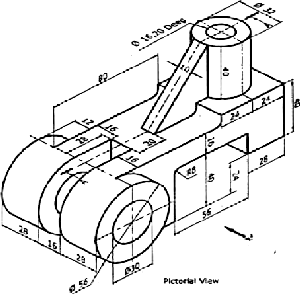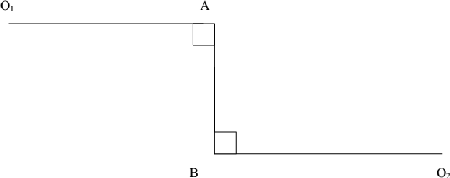MORE IN Engineering Graphics
GTU First Year Engineering (Semester 2)
Engineering Graphics
December 2011
Total marks: --
Total time: --
INSTRUCTIONS
(1) Assume appropriate data and state your reasons
(2) Marks are given to the right of every question
(3) Draw neat diagrams wherever necessary

1 (a) Explain the difference between Ist angle and IIIrd angle orthographic projection.
3 M
1 (b) Figure No.1 shows the Pictorial view of an object. Draw the following views using first angle projection method (a) Sectional Front elevation looking from direction X, take section along A-A (b) Top view (c) Side view from left. Show all dimensions11 M

2 (a) Figure No.2 shows the four bar chain mechanism O1ABO2 and the dimensions of are as below: O1A=O2B = 1125 mm connecting link AB = 375 mm. Draw the locus of midpoint M of AB, considering O1 A as driving link.7 M
2 (b) A circle of 50 mm diameter rolls along the circumference of another circle of 150 mm diameter from outside. Draw the path of a point P on the circumference of the rolling circle for one complete revolution and name the curve.
7 M

3 (a) A straight AB has its end A 10 mm above HP and end B 50 mm in front of the V.P. Draw the projections of line AB, if it is inclined to H.P. by 30° and to V.P. by 45° and it is 50 mm long.
7 M
3 (b) ABCD is a rhombus of diagonals AC = 110 mm and BD =70 mm. Its corner A is in the H.P. and the plane is inclined to H.P. such that the plan appears to be a square. The plan of diagonal AC makes an angle of 20° to the V.P. Draw the projections of the plane and find its inclination with H.P.
7 M

4 (a) The top view of 75 mm lone line AB measures 65 mm, while the length of its front view is 50 mm. Its one end A is in the H.P. and 12 mm in front of the V.P. Draw the projections of line AB and its inclinations with the H.P. and the V.P.
7 M
4 (b) The top plan of a pair of equal legs AB and AC of compass appears as an isosceles triangle having base 50 mm and vertex angle at 45° .Actual lengths of compass legs AB and AC are 120 mm. Assume points B and C on H.P. and line connecting B and C is perpendicular to V.P.. Draw the projections and find (i) the actual angle between two legs (ii) the height of point above HP and (iii) angle of plane, containing compass, makes with H.P.
7 M

5 (a) Engineering graphics is a language of all persons involved in engineering activities. Discuss the statement.
3 M
5 (b) A cone, base 75 mm diameter and axis 80 mm long is resting on its base on the H.P. It is cut by a section plane perpendicular to the V.P., inclined at 45° to the H.P. and cutting the axis at a 35 mm from the apex. Draw front view, sectional top view and true shape of the section.
11 M

6 (a) Write short note on BIS SP-46 engineering drawing standard.
3 M
6 (b) A pentagonal pyramid, side of base 40 mm and height 80 mm, is resting on its base with one of the edges of the base away from V.P. is parallel to V.P. It is cut by an AIP bisecting the axis, the distance of the section plane from the apex being 20 mm. Draw the elevation and sectional plan of pyramid and draw the true shape of the section. Also find the inclination of the section plane with the H.P.
11 M

7 (a) Define development of surfaces and state its applications in engineering field.
3 M
7 (b) A hollow square pyramid, side of base 45 mm and height 65 mm, is resting on H.P. on its base with all sides of base equally inclined to V.P. A square hole of size 20 mm is drilled through the pyramid. Sides of the square hole are equally inclined to H.P. Axis of the square hole and pyramid intersects at right angle 20mm above the base of the pyramid. Axis of the hole is perpendicular to V.P. Draw the plan, elevation and development of the lateral surfaces of the pyramid.
11 M

More question papers from Engineering Graphics